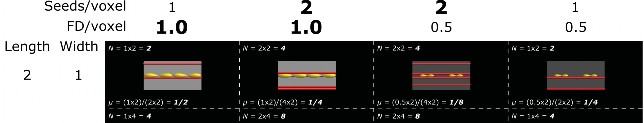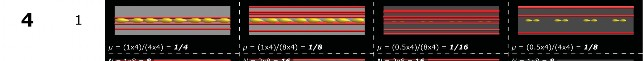# Quantitative streamlines tractography: methods and inter-subject normalisation

Robert E. Smitha,b, David Raffelta, J-Donald Tournierc, Alan Connellya,b,d

a The Florey Institute of Neuroscience and Mental Health, Heidelberg, Victoria, Australia

b Florey Department of Neuroscience and Mental Health, University of Melbourne, Melbourne, Victoria, Australia

c Centre for the Developing Brain, School of Biomedical Engineering & Imaging Sciences, King’s College London, London, UK

d Department of Medicine, Austin Health and Northern Health, University of Melbourne, Melbourne, Victoria, Australia

## ABSTRACT

Recent developments in semi-global tractogram optimisation algorithms have opened the field of diffusion magnetic resonance imaging (MRI) to the possibility of performing quantitative assessment of structural fibre ‘connectivity’. The proper application of these methods in neuroscience research has, however, been limited by a lack of awareness, understanding, or appreciation for the consequences of these methods; furthermore, particular steps necessary to use these tools in an appropriate manner to fully exploit their quantitative properties have not yet been described. This article therefore serves three purposes: to increase awareness of the fact that there are existing tools that attempt to address the well-known non-quantitative nature of streamlines counts; to illustrate why these algorithms work the way they do to yield quantitative estimates of white matter ‘connectivity’ (in the form of total intra-axonal cross-sectional area: ‘fibre bundle capacity (FBC)’); and to explain how to properly utilise these results for quantitative tractography analysis across subjects.

Keywords: Magnetic resonance imaging, diffusion, white matter, streamlines tractography, quantification

Accepted: 24.01.2022

DOI: 10.52294/ApertureNeuro.2022.2.NEOD9565

## ABBREVIATIONS

AFD: apparent fibre density;

COMMIT: convex optimisation modelling for microstructure-informed tractography; DWI: diffusion-weighted imaging (/image)

FBA: fixel-based analysis;

FBC: fibre bundle capacity (an estimate of the bandwidth of a white matter pathway); FC: fibre cross-section (NB: macroscopic change in);

FD: fibre density (microscopic);

FDC: fibre density and cross-section (combined measure of FD and FC); ‘fixel’: specific fibre population within a voxel;

FOD: fibre orientation distribution; LiFE: linear fascicle evaluation;

SIFT: spherical-deconvolution informed filtering of tractograms.

## INTRODUCTION

Since the introduction of tractography to the field of diffusion magnetic resonance imaging (MRI), there has been extensive interest in the use of this technology to assess fibre ‘connectivity’ in the brain for various neuroscientific

applications.1–4 The vast majority of tractography algorithms operate on the same fundamental mechanism: the ‘streamlines’ algorithm, where plausible white matter fibre pathways are constructed by iteratively propagating along the local estimated fibre orientation.5–9 Unfortunately, this mechanism of reconstruction does not directly facilitate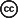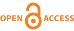Smith et al. This is an open access article distributed under the terms of the Creative Commons Attribution-NonCommercial-NoDerivs 4.0 IGO License, which permits the copy and redistribution of the material in any medium or format provided the original work and author are properly credited. In any reproduction of this article there should not be any suggestion that APERTURE NEURO or this article endorse any specific organization or products. The use of the APERTURE NEURO logo is not permitted. This notice should be preserved along with the article’s original URL. Open access logo and text by PLoS, under the Creative Commons Attribution-Share Alike 4.0 Unported license.

O R I G I N A L R E S E A R C H A R T I C L E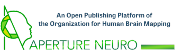O R I G I N A L R E S E A R C H A R T I C L E

one of the most fundamental parameters of interest: the density of ‘connectivity’ between two brain regions.10 A major contributing factor to this limitation is that while the streamlines algorithm enforces that the reconstructed trajectories obey the estimated orientations of the underlying fibre bundles, it provides no meaningful control over the reconstructed densities of those bundles.

The class of ‘global tractography’ methods11–15 has for many years shown promise to circumvent this problem. While in the ‘streamlines’ algorithm individual white matter trajectories are propagated independently and using only local fibre orientation information, these ‘global’ methods simultaneously solve for all connections at once, in a manner that enforces the entire tractogram reconstruction to be consistent with the raw diffusion image data. Even the most modern of these methods, however, incur considerable computational expense (particularly as reconstructions with greater numbers of connections are sought), and typically do not provide any guarantees regarding the construction of connections with biologically meaningful terminations, for instance, resulting in terminations in the white matter or cerebrospinal fluid (CSF) that are otherwise considered erroneous.16,17

A new class of ‘semi-global’ tractogram optimisation algorithms offers a potential compromise18–22; these have additionally been referred to as ‘tractogram filtering’, ‘microstructure-informed tractography’, and ‘top-down’ algorithms in various contexts. These approaches take as input a whole-brain tractogram generated using one or more streamlines tractography algorithms and modify the reconstruction in some way such that the local streamlines densities become consistent with the density of underlying fibres evidenced by the image data. These methods therefore enable quantitative assessment of fibre ‘connectivity’ (within the myriad other associated limitations of diffusion MRI and streamlines tractography), with whole-brain reconstructions that are sufficiently dense to enable higher-level analyses (e.g. connecto-mics23,24) within reasonable computational requirements. Despite the potential influence of these methods on the neuroimaging field, they have had only limited uptake. This may be due to a lack of awareness of the public availability of such methods, or a lack of understanding that these methods address some of the origins of the limitations of raw streamline count as a metric of ‘con-nectivity’. Furthermore, although these methods seek to modulate the relative connection densities of different white matter pathways within a single brain, the appropriate mechanism by which these quantities should be compared across subjects has not yet been comprehensively explained in the literature. This article therefore serves three purposes, with the aim of increasing the util-

ity of these tools in the field:

• Alert a wider audience to the fact that a primary contributing factor to the non-quantitative nature of streamlines counts can be addressed using freely available methods;

• Carefully explain and demonstrate why the design of these methods is appropriate to provide estimates of white matter connection density, including in the context of structural connectome construction;

• Explain how these estimates of connection density should be handled when performing direct comparisons between subjects.

## BACKGROUND

Before addressing the major points of this article, we first clarify the specific position and role of these ‘semi-glob-al’ tractography optimisation algorithms, the ‘connectiv-ity’ metric of interest to be derived from them, and the limitations within which they operate.

#### Requisite knowledge

The specific ‘semi-global’ methods under discussion here are intrinsically dependent on both voxel-level modelling of diffusion MRI data and streamlines tractography. As such, an adequate understanding of those concepts will be necessary for readers to follow the logic presented here; these topics are covered extensively by prior publications.2,5,9,10,25–33

#### Context and role of semi-global algorithms

Figure 1 presents the role of these methods within a tractography-based reconstruction pipeline.

• Some biological white matter bundle of interest (Figure 1a; the connection between homologous motor areas in this example) is interrogated using diffusion-weight-ed imaging (Figure 1b). Due to the sizes of the underlying axons within the white matter relative to the imaging resolution, there will typically be of the order of a million axons traversing any given image voxel.

The notion of a single scalar quantity of ‘connectivity’ of a white matter pathway is intrinsically ambiguous. If quantifying such a property of the underlying biological bundle, a reasonable interpretation would be the number of axons constituting the connection, as the information-carrying capacity of the bundle could be reasonably expected to scale in direct proportion to such. However, precisely estimating this parameter is prohibited by the limitations of diffusion-weighted imaging (DWI). The logic behind the proposed total intra-axonal cross-sectional area metric mentioned here in Figure 1 is discussed further in the ‘Metric of “connectivity”’ section.

• A diffusion model estimates from these data, within each image voxel, the orientations and densities of the fibre bundles within that voxel (Figure 1c–d).

• These orientation estimates are used by a streamlines tractography algorithm to attempt to reconstruct in

O R I G I N A L R E S E A R C H A R T I C L E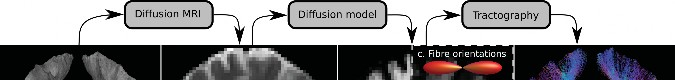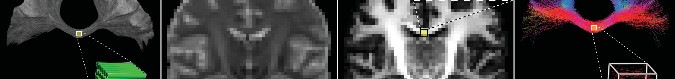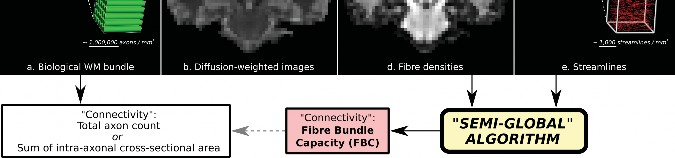Fig. 1. Contextualisation of semi-global tractogram optimisation algorithms. Given the existence of a biological white matter pathway of interest (a), diffusion-weighted imaging is performed (b). A diffusion model is fitted to these data to yield fibre orientation (c) and density (d) estimates. Fibre orientations are utilised by a tractography algorithm to produce streamlines (e). An optimisation algorithm operates on both the tractogram reconstruction and FD information to yield an estimate of bundle connectivity, here named ‘fibre bundle capacity (FBC)’, which should ideally be proportional to the connectivity of the biological bundle.

a piecewise fashion the fibres within the pathway of interest (Figure 1e). Unlike biological axons, reconstructed streamlines have no associated volume and are therefore shown as infinitesimally thin in Figure 1e. The number of streamlines traversing any given image voxel may be of the order of 1,000, but varies wildly depending on reconstruction parameters.

• The role of such a ‘semi-global’ tractogram optimisation algorithm is to combine the reconstructed tractogram with fibre density (FD) information from the diffusion model (or alternatively the diffusion-weight-ed image data themselves; see the ‘Comparing tractograms and image data’ section), relying on the quantitative nature of these FD estimates to overcome the non-quantitative nature of streamlines tractography.

• The outcome of such a process is a derived measure of ‘connectivity’ of the pathway of interest (here named ‘fibre bundle capacity (FBC)’; more in the ‘Metric of “connectivity”’ section). If calculated appropriately, this measure should be a reasonable proxy for the information-carrying capacity of the biological pathway.

Unlike other analysis techniques that interrogate the values of quantitative properties as they vary along the length of a white matter bundle of interest,34–39 here the derived experimental output is a single scalar measure reflecting the total ‘connectivity’ of that pathway between its two endpoints.

construction and subsequent interrogation of the brain ‘structural connectome’.23,24 Within this framework, a parcellation of the grey matter is defined, and for every possible unique pair of grey matter regions, a scalar measure of ‘connectivity’ is quantified, with these values together forming a connectivity matrix that encodes the value of this connectivity metric between pairs of regions in their corresponding rows/columns.29,30,40 Such connectome construction can therefore be thought of as simply repeating this quantification process many times, where each ‘bundle of interest’ is defined based on the streamline endpoints being ascribed to a specific pair of grey matter regions. So, in the context of connectomics, the techniques described here for characterising such ‘con-nectivity’ are intended to:

• Supersede the use of streamline count, which continues to be used in neuroscientific applications despite being known to be biased by many reconstruction-re-lated parameters10,19,41;

• Provide a measure of ‘connectivity’ for which, when applying higher-order analyses that implicitly interpret such data as the bandwidth of information flow around a network,42,43 such an interpretation is more direct and intuitive than alternative measures such as aggregate microstructural quantities.

#### Metric of ‘connectivity’

The notion of ‘connectivity’ in the context of diffusion MRI tractography remains ambiguous without a very explicit description of exactly what metric is derived from

O R I G I N A L R E S E A R C H A R T I C L E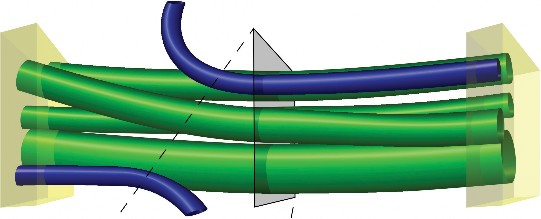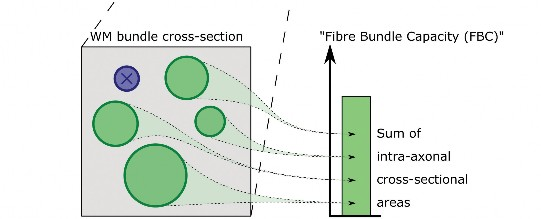Fig. 2. Visual depiction of the fibre bundle capacity (FBC) metric. A white matter bundle of interest is defined based on its endpoints, shown as yellow cuboids. Only those fibres that are attributed to both endpoints are constituent members of that bundle (green cylinders). The FBC is defined as the sum of the intra-axonal cross-sectional areas of these fibres.

the image data/tractogram reconstruction. In the diffusion MRI tractography literature, myriad metrics have been utilised, all of which have been referred to at some point as simply ‘connectivity’.

In the context of the methods discussed here, our target scalar metric of interest when quantifying ‘white matter connectivity’ is the total fibre cross-sectional area of a fibre bundle (ideally, the intra-axonal cross-sectional area). The nature of this metric is presented visually in Figure 2, where a bundle of interest is defined based on those fibres connecting two endpoints of interest, and the intra-axonal cross-sectional areas of only those fibres attributed to the bundle of interest are summed to derive this estimate. This metric has previously been shown to converge white matter connection density estimates towards gross axon count estimates from post mortem dis-section.44 To facilitate discussion of higher-level concepts in the context of this metric, we henceforth refer to this metric as the ‘FBC’. This term communicates that the intent of this measure is the capacity of a white matter fibre bundle to transmit information between its endpoints. Ideally, derivation of this measure should be as sensitive and specific as possible to the intra-axonal cross-section-al area of the biological fibres constituting the pathway

being reconstructed. While for simplicity this metric can be thought of as a proportional estimate of axon count, the precise attributes of this metric are discussed later in the ‘Qualifying the “Fibre Bundle Capacity (FBC)” met-ric’ section.

The important distinction between FD estimates quantified at the voxel level, and fibre connection density estimates (i.e. FBC) quantified at the level of pathways of interest, is demonstrated in Figure 3. There are various diffusion models that include some parameters related to fibre volume for each image voxel (Figure 3a–d). In the context of this article, however, we seek to quantify the total fibre cross-sectional area associated with some specific pathway of interest (Figure 3e–f).

#### Limitations of semi-global optimisation algorithms

• We do not consider the sub-voxel spatial configurations of fibre bundles in either the image45 or tracto-gram46 domains; we consider only that each voxel is the sum of its constituent parts, irrespective of sub-voxel position;

O R I G I N A L R E S E A R C H A R T I C L E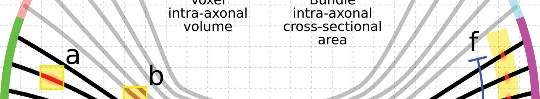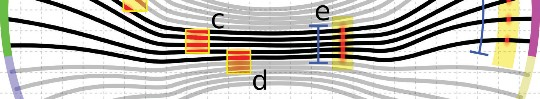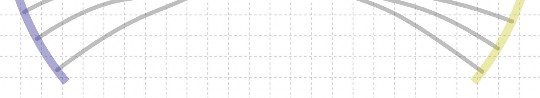Fig. 3. Relationship between quantification of intra-axonal volume within individual voxels (FD), and quantification of intra-axonal cross-sectional area of a white matter bundle of interest (fibre bundle capacity (FBC)). A bundle of interest is defined based on selection of two parcels (yellow surfaces) within a grey matter surface segmentation (planes at far left and far right of the figure). (a) A diffusion model may provide, within each individual image voxel, an estimate of intra-axonal volume (FD). Any bundle of interest will likely traverse a large number of image voxels along its length and breadth. (b) The parameter of interest for quantifying the ‘connectivity’ of this white matter pathway is the total intra-axonal cross-sectional area of the axons attributed to the bundle of interest (FBC). Note that this is irrespective of the length of the pathway or the total dimensions of the plane necessary to encapsulate all axons within that pathway.

• We assume that the diffusion signal measured in a voxel is the sum of signal contributions from the matter fibre bundles and other tissues within that volume (i.e. the ‘slow exchange’ regime);

• We do not consider influencing streamlines trajectories based on microstructural information, as discussed47 and proposed48 recently; we consider only the use of microstructural/image information to modulate the reconstructed densities of different white matter pathways.

#### Comparing tractograms and image data

Throughout this discussion, we use the term ‘fixel49 to refer to a specific fibre population within a particular voxel. Each voxel in the diffusion image may contain multiple fixels (‘crossing fibres’), and the number of fixels may vary between different voxels. Use of such terminology assists in disambiguating this concept from a macroscopic white matter fascicle that connects two areas of grey matter, each of which will be associated with many fixels along its length and breadth.

Using diffusion image data to provide a tractogram with quantitative attributes requires a mechanism by

which to compare the tractogram to those image data. There are two principal mechanisms by which this may be done (demonstrated in Figure 4):

1. Each streamline contributes some intensity to the reconstructed diffusion signal based on a forward model. Typically, a diffusion tensor with fixed diffusivi-ties is chosen. Signal intensity from sources other than white matter fibres may additionally be modelled as isotropic or anisotropic sources contributing to the diffusion signal and included in the optimisation. The reconstructed image data from the tractogram (and possibly other tissue sources) is compared directly to the empirical diffusion data.

2. The white matter fibre density within each fixel is first estimated based on an inverse model, potentially with estimation and separation of other signal sources (e.g. other tissues or fluid).50–58 Here, we focus on the spherical deconvolution model,31,59,60 though other approaches can certainly be used. The reconstructed streamlines density from the tractogram ascribed to each fixel is compared directly to the corresponding white matter fibre densities estimated from the diffusion model.

O R I G I N A L R E S E A R C H A R T I C L E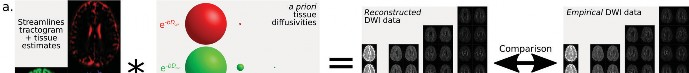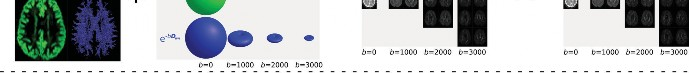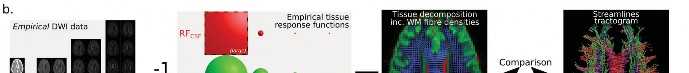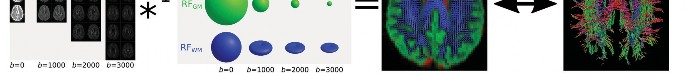Fig. 4. Relationship between two different modelling approaches used in ‘semi-global’ tractography optimisation algorithms. (a) Based on estimates of local densities of different types of tissue (including orientation information in the case of white matter streamlines), and functions describing how each tissue contributes to the diffusion signal at different b-values (often derived from e.g. the diffusion tensor model), a spherical convolution is performed to estimate the diffusion signal from the current tractogram reconstruction. This is compared to the empirical diffusion signal intensity data, and the tissue density estimates within the reconstruction are revised accordingly. (b) Based on tissue response functions describing the appearance of each type of tissue in the diffusion data (determined either from some model or from the image data directly), a spherical deconvolution is performed to obtain estimates of tissue densities (including orientation information in the case of white matter fibres). The densities of a reconstructed tractogram are compared to the white matter fibre density estimates, and the parameters of the tractogram are revised accordingly.

Although the former approach is more ‘conventional’, and additionally has a long history of use in the context of global tractography methods, for demonstration purposes we use the latter model, as it provides a more intuitive course of reasoning in the following sections.

Note that these two approaches are directly related via the invertibility of the spherical convolution transform: the a priori definition of the forward models to be used for each tissue component/compartment in approach (1) serves the same purpose as the a priori definition of the tissue ‘response functions’ for spherical deconvolution in approach (2) (Figure 4). Note also that in Figure 1, the ‘semi-global algorithm’ is shown to be utilising information from estimated fibre densities rather than the diffusion-weighted images, corresponding to case (2) described here.

There is an important difference between these two cases that is requisite for proper understanding of subsequent sections of this article. In mechanism (1), the model considers not only the density of streamlines within any particular image voxel, but also the precise orientation distribution of those streamlines: the contribution of each streamline towards the reconstructed diffusion-weight-ed signal is based on the tangent of the streamline at each location along its trajectory. In mechanism (2), the diffusion model defines a small finite number of discrete fixels in each image voxel; the algorithm that maps each streamline to the DWI voxel grid19 determines the orientation of each streamline-voxel intersection, which is

then attributed to the appropriate fixel within that voxel. As such, each streamline has associated with it a set of fixels traversed, and each fixel has associated with it both an FD as estimated by the diffusion model and a total streamlines density based on the set of streamlines traversing it. The algorithms described below involve direct utilisation of this per-fixel information (to a greater or lesser extent). While mechanism (1) is potentially sensitive to fibre/streamlines orientation distribution information that is more complex than what can be represented using a small finite number of discrete fixels in each voxel, mechanism (2) essentially utilises the fixels provided by the diffusion model as a sparsifying transform, reducing the size of the computational problem; further, the fact that it is possible to perform for each fixel a direct scalar comparison between FD and total streamlines density makes this mechanism more amenable to promoting an understanding of the logic underlying the methods described in this article.

## METHODS

#### The algorithmic basis of quantitative streamlines tractography

In order to demonstrate the fundamental operation of the algorithms under discussion (and hence the quantitative properties they provide), we begin with a simple

definition of the fundamental data and research question that may be applicable to an example analysis involving diffusion MRI tractography.

• What we want:

• An estimate of the FBC metric for some pathway, as defined in the Background section and demonstrated in Figures 2 and 3.

• What we have:

• A measure of fibre volume for each fixel as estimated via a diffusion model;

• A set of streamlines delineating the trajectory of the pathway of interest, typically based on a priori regions of interest or other criteria to isolate the pathway;

• A whole-brain tractogram, of which the set of streamlines ascribed to the pathway of interest is a subset (while this is not required for Algorithm 1, its necessity will be demonstrated in later algorithms).

The example to be used for demonstration in this article is the connection between left and right precentral sulci, as derived from the ‘Desikan-Killiany’ parcellation61 provided by the FreeSurfer software62; this is shown in Figure 5.

We now demonstrate in this section several plausible algorithmic approaches by which our goal may be achieved. We start by proposing a relatively simple and naïve algorithm, observing its benefits and shortcomings, and then use these observations to derive increasingly advanced approaches, eventually presenting a total of four algorithms.

Algorithm 1 is the simplest possible approach for incorporating the FD information from a diffusion model into estimating FBC for a pathway of interest reconstructed using streamlines tractography. It is based on the observation

O R I G I N A L R E S E A R C H A R T I C L E

that a pathway volume can be derived by computing a mask of fixels that are traversed by the streamlines belonging to that pathway, and this can be converted to a pathway cross-sectional area based on the length of the pathway (Figure 6; see also pseudocode in Appendix):

Step 1. Identify all fixels that are traversed by at least one streamline belonging to the pathway.

Step 2. Sum the fibre volumes of all selected fixels to calculate the total fibre volume of the pathway.

Step 3. Divide this value by the mean streamline length to estimate FBC for the pathway.

Figure 7a shows the assignment of FD from the underlying diffusion model field to the quantification of volume (and hence cross-sectional area) of this specific pathway. There are a couple of weaknesses in this algorithm observed in Figures 6 and 7a:

• The effect of ‘outlier streamlines’: those streamlines that are attributed to the pathway of interest but follow a trajectory drastically different from the rest of the streamlines assigned to the pathway. When this occurs, all of the fixels traversed by that streamline are added to the fixel mask, and all of the FD within each of those fixels contributes to the total fibre volume of the pathway. One or a small number of stray streamlines may therefore drastically increase the final quantification of FBC (e.g. the streamline in Figure 5 that travels partway down the corticospinal tracts as it traverses between the two regions of interest).

Utilisation of some more stringent criteria for inclusion of fixels in the mask (e.g. an increased streamline count threshold) could theoretically mitigate this effect, though what form such criteria should take is subjective. Alternatively, such errors may be addressed using additional tractography regions-of-interest or manual quality control procedures; but such mitigation does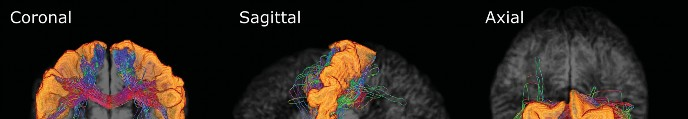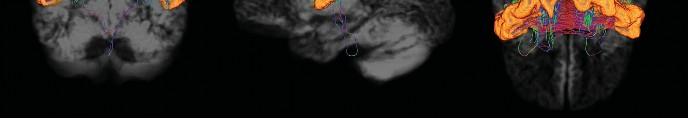Fig. 5. Visualisation of the pathway of interest to be used in a demonstration of the four fibre bundle capacity (FBC) quantification algorithms presented in the ‘Methods’ section: three orthogonal views. The left and right precentral sulci are highlighted in orange; streamlines are coloured according to their local tangent orientation (red = left-right; green = anterior-posterior; blue = inferior-superior).

O R I G I N A L R E S E A R C H A R T I C L E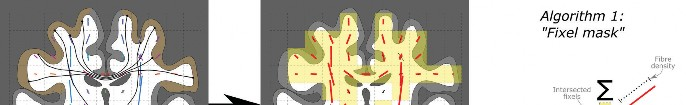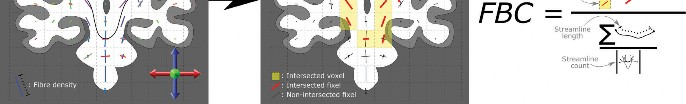Fig. 6. Visual demonstration of the operation of Algorithm 1 (‘Fixel mask’). For a particular pathway of interest (left panel: grey matter regions labelled orange, leading to selection of streamlines shown), those fixels traversed by the streamlines corresponding to that pathway are selected (right panel: red fixels within yellow voxels). The sum of the microscopic fibre densities of these selected fixels (equation numerator; encoded visually as fixel lengths) is divided by the mean streamline length (equation denominator: sum of streamline lengths divided by the number of streamlines) to yield the fibre bundle capacity (FBC) measure.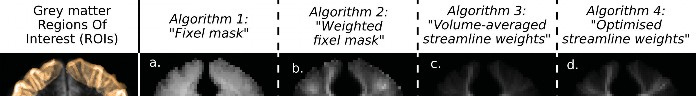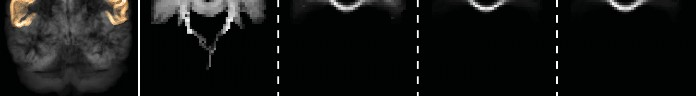Fig. 7. (Left) Coronal projection of brain grey matter, with regions of interest used in the reconstruction of the pathway of interest highlighted; (a–d) Maximum intensity projection (MIP) spatial distributions of the density of white matter fibres attributed to the pathway of interest resulting from quantification using the four algorithms described in the ‘Methods’ section. The reconstructed bundle is that shown in Figure 5.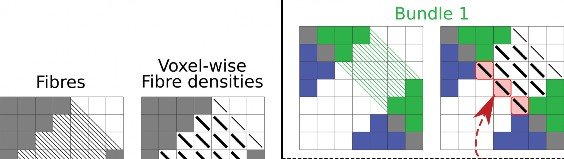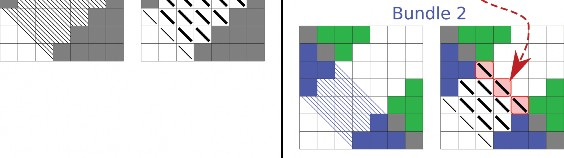Fig. 8. The effects of partial volume on Algorithm 1. Left: A white matter fibre pathway connecting between two grey matter regions, shown as both fibre trajectories and per-voxel fibre orientation/density. Right: The pathway is split into two bundles of interest based on parcellation of the voxels at the endpoints of the pathway; a subset of voxels (highlighted red) is intersected by both bundles.

not trivially extend to studies where many different pathways are assessed (e.g. when building the structural connectome over the whole brain).

• The local FD per voxel attributed to the pathway is relatively consistent throughout the entire pathway, from the corpus callosum through the centrum semiovale and to the interface between grey and white matter. This is, however, contrary to how such tracts are constructed physically: as white matter fibres fan out from the narrow cross-section of the corpus callosum to a long strip of grey matter, the local voxel-wise density of the fibres within this specific pathway would be expected to decrease.

The way in which these effects can manifest, as well as the source of the limitation, is demonstrated in Figure 8. Here the selection of two bundles of interest from a larger white matter pathway is shown, both in the corresponding streamlines and in the fixels to which they are ascribed. What is highlighted in red is the fact that if each of the two bundles is independently mapped to the corresponding voxels traversed, then for the set of voxels intersected by both bundles, all of the FD within those voxels will be attributed to both bundles. This has two effects: firstly, the spatial distribution of FD attributed to each bundle individually does not vary smoothly, failing to represent partial volume at the outer edge of each bundle similarly to that observed in Figure 7a; secondly, the sum of the calculated fibre connectivity of the two bundles would be greater than that of the actual white matter structure — without even necessitating tractography reconstruction errors — as the FD in those voxels would contribute to the quantification of both bundles.

Algorithm 2 directly addresses the major imperfections of Algorithm 1 that arise due to partial volume effects.

O R I G I N A L R E S E A R C H A R T I C L E

It is based on the observation that when a fixel is traversed by streamlines belonging to the pathway of interest in addition to other streamlines not belonging to that pathway, then rather than the entire fibre volume of that fixel contributing to the pathway, ideally only the fraction of that fixel attributed to the pathway of interest should be included. This is achieved as follows (Figure 9; see also pseudocode in Appendix):

Step 1. Generate a whole-brain tractogram; define the pathway of interest as a subset of those whole-brain streamlines.

Step 2. For every fixel, calculate the fraction of the total streamlines density in that fixel that belongs to the pathway of interest.

Step 3. The contribution of the fibre volume of each fixel to the fibre volume of the pathway of interest is modulated by the fraction of that fixel ascribed to the pathway of interest in step 2.

Step 4. As in Algorithm 1, divide the total volume of the pathway by the mean streamline length to estimate FBC.

The primary advantage of this approach over Algorithm 1 is that if a fixel is only traversed by a small number of streamlines within the pathway of interest, that fixel only contributes a small amount of its fibre volume to the FBC result. The effect of this change from Algorithm 1 is particularly evident in the inferior half of the brain in Figure 7b, where individual erroneous streamlines trajectories contribute far less to the calculated fibre pathway volume.

While various streamline reconstruction biases mean that the fixel fibre volume fractions ascribed to the streamlines within the pathway of interest may not be precisely equivalent to the total fraction of the underlying fibres within that fixel that belong to the biological pathway of interest, this algorithm certainly provides a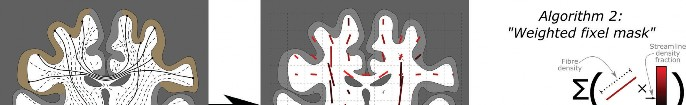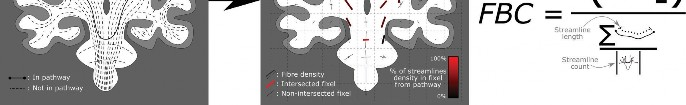Fig. 9. Visual demonstration of the operation of Algorithm 2 (‘Weighted fixel mask’). The streamlines corresponding to the pathway of interest (left panel: solid lines) are a subset of a whole-brain tractogram (left panel: dashed lines). For each fixel in the image (right panel), the fraction of the streamlines density in that fixel corresponding to the pathway of interest can be quantified (right panel: red intensity). The contribution of the fibre volume within each fixel (encoded visually as fixel lengths) to the pathway of interest is modulated by the fraction of the streamlines density in that fixel attributed to the pathway of interest (multiplication in equation numerator); as with Algorithm 1, this volume is then divided by the mean streamline length (equation denominator: sum of streamline lengths divided by the number of streamlines) in the calculation of fibre bundle capacity (FBC).

O R I G I N A L R E S E A R C H A R T I C L E

perceptible improvement over Algorithm 1, with the spatial distribution of fibre volume within the pathway having a much more biologically plausible appearance.

An inherent problematic issue in the design of Algorithm 2, however, is that it fails to enforce a consistent intra-axonal cross-sectional area within the pathway. Along the length of the fibre bundle shown in Figure 7b, there are local ‘hot-spots’ of supposedly increased fibre volume, both within the superficial white matter and where the bundle intersects the grey matter targets. While individual axons may have some modulation in their diameter along their length, such gross modulation of macroscopic intra-axonal cross-sectional area is physically unrealistic for white matter pathways at the macro-scale. Observation of such in diffusion MRI data is therefore far more likely to be an artefact of image analysis and reconstruction. Furthermore, at the endpoints of the pathway, voxels containing partial volume between grey and white matter are likely to contain a smaller number of streamlines than those voxels entirely within the white matter, which results in the fraction of fixel FD assigned to the pathway of interest being prone to quantisation effects; this contributes to the ‘speckly’ appearance of the density map in Figure 7b near the grey matter.

Algorithm 3: ‘Volume-averaged streamline weights’

In order to overcome the fundamental limitation of Algorithm 2, a solution is sought for deriving FBC that enforces a constant intra-axonal cross-sectional area along the length of the pathway. We clarify here that this constraint does not apply to the macroscopic span of space traversed by fibres of that bundle, but applies specifically to the intra-axonal portion of the bundle. For example: when fibres within a tightly packed bundle diverge

from one another (commonly referred to as ‘fanning’), the surface area of the subset of a plane encapsulating all fibres in the bundle may increase (the ‘macroscopic cross-section’), but the sum of intra-axonal cross-sectional areas should remain unchanged if the axon diameters are consistent along their length (e.g. Figure 2; Figure 3e–f); here it is the latter definition that we advocate should be constrained.

Algorithm 3 is an initial realisation of this concept. It enforces constant intra-axonal cross-sectional area of the pathway, by requiring that each streamline in the pathway contributes a constant fibre cross-sectional area along its entire length. Hence, unlike Algorithms 1 and 2, here contributions towards FBC are made not per fixel, but per streamline (in the context of semi-global tractography algorithms, these parameters are sometimes referred to as ‘weights’).

This algorithm operates as follows (Figure 10; see also pseudocode in Appendix):

Step 1. Using the whole-brain tractogram, calculate the total streamlines density in each fixel.

Step 2. For each streamline, calculate the fibre volume to be attributed to that streamline. Every fixel traversed by the streamline contributes a fraction of its fibre volume to the sum for that streamline, based on the fraction of the total streamlines density in that fixel that was contributed by that particular streamline.

Step 3. Convert the fibre volume of each streamline to a fibre cross-sectional area, by dividing by the length of that streamline.

Step 4. Sum the cross-sectional areas of the streamlines belonging to the pathway of interest to derive FBC.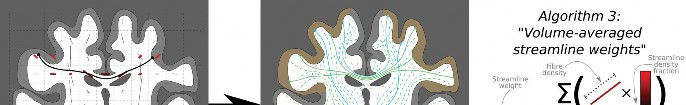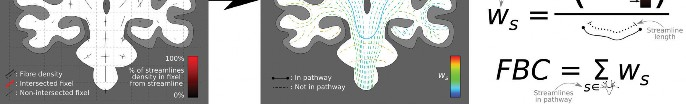Fig. 10. Visual demonstration of the operation of Algorithm 3 (‘Volume-averaged streamline weights). For each individual streamline, the total fibre volume attributed to that streamline (numerator, first equation) is based on the products of the fixel fibre volumes (encoded visually as fixel lengths) and the fraction of the streamlines density in each of those fixels attributed to the streamline of interest (left panel: encoded as red intensity); this fibre volume is then divided by the length

of that individual streamline (denominator, first equation) to ascribe a ‘weight’ ws to each individual streamline s (right panel: streamline colours). The fibre bundle

capacity (FBC) of the pathway of interest is then the sum of the weights ascribed to the streamlines attributed to the pathway of interest (right panel: solid lines).

While this algorithm produces a measure of fibre cross-sectional area per streamline rather than fibre volume per fixel, it is still possible to reconstruct the latter; this allows us to generate a spatial map of FD attributed to the pathway of interest that can be compared to Algorithms 1 and 2. The product of a cross-sectional area with a length yields a measure of volume; hence, each streamline in the tractogram contributes a fibre volume to every voxel it traverses, based on the product of its weight and the length of the streamline segment that intersects that voxel. The result of this process is shown in Figure 7c. Compared to the previous two algorithms, this approach produces an FD map for the resulting pathway that appears quite biologically reasonable, with a maximal microscopic FD within the narrow confines of the corpus callosum that decreases as those fibres fan out towards the cortex.

There does, however, remain one slight inadequacy with this algorithm. Consider an experiment where, instead of deriving a spatial map of reconstructed fibre volume for a particular pathway of interest only (as we have been doing here), we instead map the spatial distribution of reconstructed fibre volume of the entire tractogram. If the streamlines weights are faithful to the intra-axonal cross-sectional areas of the biological fibres following the trajectories reconstructed by those streamlines, then one would expect an accurate reproduction of the fibre volumes that were estimated from the voxel-wise diffusion model throughout the white matter (or equivalently: applying the forward model to the whole-brain tractogram should yield the empirical diffusion signal, as demonstrated in the ‘Comparing tractograms and image data’ section and Figure 3). Note this process is very similar to track density imaging (TDI) at native DWI resolution,63–65 incorporating the ability for streamlines to contribute differentially towards the image.

This experiment is shown in Figure 11. The reconstructed FD from the outcome of Algorithm 3 (Figure 11c) is closer to the voxel-wise estimate of white matter

O R I G I N A L R E S E A R C H A R T I C L E

FD derived from spherical deconvolution (Figure 11a) than the original tractogram where every streamline contributes equally (Figure 11b). However, it still does not provide an entirely faithful representation of the underlying white matter FD field. This is perhaps expected given the nature of the algorithm itself. From a physical perspective, the operation of this algorithm can be thought of as taking the sum of fibre volumes attributed to the streamline by each fixel (which may be greater or lesser at different points along the streamline) and spreading this fibre volume evenly along the length of the streamline in order to ascribe to it a constant fibre cross-sectional area. While this process does go some way to incorporating fibre volume information from the diffusion model into the tractogram, it fails to directly enforce consistency between the estimated FD and reconstructed streamlines density for each fixel of the image (the residual discrepancy visible between Figure 11a and 11c). In the case of the specific pathway of interest used in this demonstration, the FD within the corpus callosum projected by the tractogram is clearly greater than that indicated by the image data; this means that the calculated FBC for this connection relative to other pathways when using Algorithm 3 would likely be an over-estimate.

Algorithm 4: ‘Optimised streamline weights’

Addressing the remaining problem with the approach described in Algorithm 3 — the fact that the fibre volumes estimated from the tractogram are not a sufficiently accurate reconstruction of the fibre volumes estimated from the diffusion model, as shown in Figure 11 — is a fundamental requirement if we are to consider the streamline weights truly quantitative. If the streamlines trajectories and ascribed weights are reflective of the underlying biological connectivity, then the spatial distribution of FD throughout the white matter represented within this connectivity-based reconstruction should accurately match estimates of this measure that are derived from the image data directly.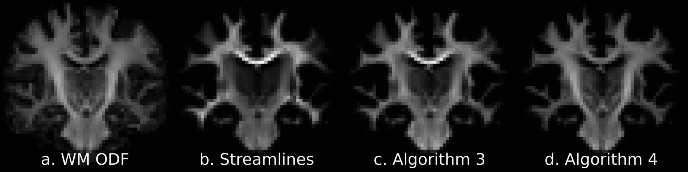Fig. 11. Comparison of spatial distributions of track densities from whole-brain tractogram data with the density of white matter fibres as estimated through spherical deconvolution. (a) The orientationally averaged mean of the white matter orientation distribution functions (the l=0 term of the spherical harmonic expansion) as a measure of total fibre density within each voxel. The distribution of TD within a whole-brain tractogram should ideally match these data. (b–d) The density of streamlines in the whole-brain tractogram when the contribution of each streamline to the map is modulated as follows: (b) no modulation (all streamlines contribute equally); (c) modulated by the weights ascribed to the streamlines by Algorithm 3 (‘Volume-averaged streamline weights’); (d) modulated by the weights ascribed to the streamlines by Algorithm 4 (‘Optimised streamline weights’).

O R I G I N A L R E S E A R C H A R T I C L E

This limitation is addressed by designing an algorithm that explicitly seeks to derive a set of streamline weights that result in an accurate reconstruction of the underlying fibre volumes estimated from the diffusion model (or equivalently, an accurate reconstruction of the empirical diffusion signal using a forward model). This basic concept is shown diagrammatically in Figure 12 and might proceed, for example, as follows (see also pseudocode in Appendix):

Step 1. Initially assign a unity weight to each streamline. Step 2. Using the whole-brain tractogram, based on the current streamline weights, calculate the total

streamlines density in each fixel.

Step 3. For each fixel, calculate the difference between the FD estimated from the diffusion model and the total attributed streamlines density.

Step 4. For each streamline, increase or decrease the weight in order to minimise the error quantified in step 3.

Step 5. Loop back to Step 2 until some termination criterion is met.

Step 6. Sum the weights of those streamlines belonging to the pathway of interest to derive FBC.

This is the mechanism by which the ‘spherical-deconvolution informed filtering of tractograms 2 (SIFT2)’ method22 operates. The spatial distribution of FD within the pathway of interest after having applied the SIFT2 algorithm is shown in Figure 7d, and the spatial

distribution of FD of the entire tractogram is shown in Figure 11d. Crucially, the latter demonstrates a highly accurate reproduction of the underlying FD field estimated from the diffusion model (Figure 11a), highlighting how this approach provides a tractogram-based connectivity model that obeys the fundamental spatial constraints imposed by the physical nature of the underlying biological fibre structure. It is this observation that permits the streamline weights estimated by such algorithms to be used in the quantification of FBC (within the constraints imposed by other limitations associated with diffusion MRI streamlines tractography66).

#### Inter-subject connection density normalisation

Whenever quantitative data are to be compared across subjects, an important distinction must be made between absolute and relative quantitative measures. For instance, when assessing the fractional anisotropy (FA) measure67 from the diffusion tensor model,68 there is no need to modulate these values differentially between subjects, as it is an absolute measure (for any given DWI acquisition scheme) and therefore any differences in this metric between individuals can be interpreted according to the properties encapsulated within that metric. However, if a quantitative measure is relative to other latent parameters that vary across individuals, care must be taken to appropriately handle the effects of those latent parameters, in order to be able to interpret any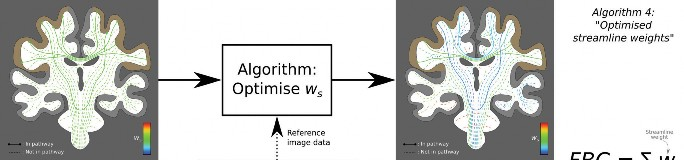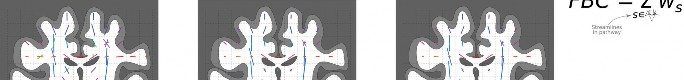Fig. 12. Visual demonstration of the operation of Algorithm 4 (‘Optimised streamline weights’). For a whole-brain tractogram (top left panel), the total streamlines density traversing each fixel (bottom left panel; encoded visually as fixel lengths) may not match the fibre volumes estimated from the diffusion model (bottom panel; fixel lengths). This algorithm modulates the weight ws ascribed to each streamline s (top right panel: streamline colours) in order to achieve correspondence between the total streamlines density traversing each fixel (bottom right; fixel lengths) and the diffusion model fibre density estimate (bottom panel). The fibre bundle capacity (FBC) measure for the pathway of interest is the sum of the weights ascribed to those streamlines attributed to the pathway of interest (top right panel; solid lines only; streamline colours).

differences in that metric as being specific to that metric rather than some nuisance confound.

In the fixel-based analysis (FBA) framework,69 which enables statistical analysis of white matter quantitative measures in the presence of crossing fibres, FD estimates must be comparable across subjects throughout some common template space. In the context of apparent fibre density (AFD) quantification70 using the spherical deconvolution model,59 the fibre orientation distributions (FODs) (i.e. the representation of estimated fibre directions and densities in each voxel) are deliberately not normalised either to a unit integral in each voxel or to the intensity of the b=0 image (i.e. volume acquired with no diffusion sensitisation) in each voxel. This makes the size of the FOD directly proportional to the magnitude of the DWI signal (which is itself proportional to intra-cellu-lar volume at high b-values70); the size of the FOD is also inversely proportional to the magnitude of the response function used for deconvolution70:

FOD RF= DWI FOD = DWI 1RF (1)

(‘RF’: response function; ‘*’: convolution operation; ‘*−1’: deconvolution operation)

Enabling direct comparison of this measure across subjects therefore necessitates global inter-subject intensity normalisation, in order for AFD to be minimally influenced by nuisance variables. This typically includes B1 bias field correction, scaling of DWI intensities to a

O R I G I N A L R E S E A R C H A R T I C L E

incontrovertible, it is in fact an imperfect solution to connection density normalisation. By using a fixed number of streamlines per subject, each streamline effectively represents a fixed, subject-specific fraction of the total white matter fibre connectivity. While comparing such quantitative measures across subjects is acceptable as long as they are properly interpreted as such, this metric fails to take into account a number of factors that may differ between subjects that may correspondingly introduce biases or unwanted variance into such an analysis; this includes both biological differences (e.g. widespread reductions in FD) and features of the tractogram reconstructions (e.g. differences in streamline lengths). For a genuine quantitative comparison of absolute fibre connection densities between subjects, greater care must therefore be taken.

Here, we demonstrate our recommendation for how this normalisation should be achieved when using specifically the model underlying the ‘SIFT’19 and ‘SIFT2’22 methods, which is itself directly dependent on the AFD measure. Use of alternative reconstruction techniques (in terms of either the underlying diffusion model or alternative semi-global tractography methods) would require that appropriate comparable steps be taken.

The SIFT model defines the proportionality coefficient μi for subject i, which relates the global sum of track density (TD) to the global sum of estimated FD in the single-subject reconstruction, computed across all fixels f in that subject:

common intensity value according to some representa-

µ = fεi FDf

(2)

tive image statistic (e.g. mean b=0 magnitude in white

matter), and use of a group average response function for deconvolution70: these together ensure that ‘one unit of AFD’ is comparable across subjects, rather than being defined relative to subject-specific parameters (e.g. coil loading, scanner receiver gain, subject-specific response function magnitudes).

In a similar manner, quantitative analysis of FBC requires inter-subject connection density normalisation: that is, if we quantify the intra-axonal cross-sectional area of a particular pathway (e.g. edge of a connectome) across multiple subjects, we want these quantities to be directly comparable across subjects, without being biased by confounding factors that destroy the physical interpretation of this measure or introduce substantial correlations with nuisance reconstruction parameters.

In the brief history of diffusion MRI tractography connectomics, this normalisation has most commonly been achieved by simply generating the same number of streamlines for each subject. Or, expressed in an alternative way: if half as many streamlines were generated for one subject as there were for all other subjects, it would seem intuitively logical that the streamline counts in each edge for that subject should be doubled in order for the raw values stored in the connectome matrices to be comparable to other subjects. While generation of an identical number of streamlines across subjects may seem

i fεi TD

f

(For simplicity, the influence of the processing mask within the SIFT model19 is omitted here.)

In the original SIFT method, this parameter permits direct comparison between the streamlines density and fibre volume within each fixel, in order to drive the streamlines filtering process. In SIFT2, it approximately centres the distribution of streamlines weights about unity. Note that all parameters within this expression are subject-specific.

As FD is a measure of volume (dimensions L3) and TD is a sum of streamline lengths (dimensions L), µ is a measure of cross-sectional area, with dimensions L2. For every streamline, this parameter (multiplied by the weight assigned to that streamline in the case of SIFT2) is a measure of the intra-axonal cross-sectional area represented by that streamline. For each voxel traversed by the streamline, the product of this cross-sectional area with the length of the streamline intersection within that voxel produces the fibre volume contributed to that fixel by that particular streamline within the model.

In the context of FBC quantification, we are interested not in these fixel-wise fibre volumes, but the connection densities of specific macroscopic pathways of interest. Any such pathway is represented as a subset of streamlines in the whole-brain tractogram. For an example

O R I G I N A L R E S E A R C H A R T I C L E

pathway p (which is reconstructed by a subset of streamlines s), it is the sum of intra-axonal cross-sectional areas of the streamlines within that pathway that gives a measure of the intra-axonal cross-sectional area of the pathway FBCp,i:

The global intensity normalisation and group average response function components of the recommended pre-processing pipeline for AFD analysis are tailored to make equivalent across subjects the values of DWIref and AFDref, respectively. As such, if this pipeline is followed,

FBC = µ . W

(3)

the term AFDref

is identical across subjects by construc-

p,i

i Siεp

i

DWI

ref

s

For subject i, the connection density FBCp,i of pathway p is the product of the subject-specific proportionality coefficient µi and the sum of streamline weights wi of those streamlines si belonging to pathway p.

(Note that in the original SIFT method, ws = 1 for all

retained streamlines after filtering, but µi is modulated during the filtering process.)

This equation suggests that if one wants to compare FBC across subjects (whether for an individual bundle of interest or an entire connectome matrix), simply multiplying the sum of bundle streamlines weights by μi is sufficient to produce a measure of FBC that can be compared across subjects. However, parameter μi only considers the fibre densities and track densities within a single subject; in order to compare these quantities between subjects, we must ensure that parameter μi is adjusted appropriately to account for between-subject variation, by ensuring that the fundamental scaling underlying this parameter is equivalent between subjects.

We can extend Equation (2) as follows:

tion, and simply multiplying the sum of streamline weights within a pathway of interest (e.g. a connectome edge) by μi permits direct quantitative comparison of FBC between subjects, in a manner that appropriately accounts for many variables that would otherwise confound the interpretation of streamline counts as ‘connection density’.

The consequences of this connection density normalisation are demonstrated in Figure 13. This demonstration consists of 16 individual synthetic subjects, each of whom possesses a single white matter fibre bundle. The fundamental properties of this bundle — length, width, and microscopic FD per voxel — vary among the subjects; additionally, the number of streamlines seeded in each white matter voxel is also varied. The lower part of Figure 13 then demonstrates visually how the quantified ‘connectivity’ of this bundle across the 16 subjects changes in magnitude across the different subjects, depending on the exact measure of ‘connectivity’ that is utilised. The connectivity measures demonstrated are as follows:

=  AFDref . x y DWI ref

z .  fεi FDf

fεi TDf

(4)

• The macroscopic bundle volume V;

• The streamline count N;

• The number of streamlines divided by the length of the streamlines41,71;

• μi,adj is the proportionality coefficient of subject i ‘ad-justed’ for facilitation of inter-subject comparison;

• The first term,  AFDref , is specific to the spherical de-

DWI ref

convolution model if processing were to be performed independently for each subject. It relates to the global scaling of AFD magnitudes within that subject, which is dependent on the magnitude of the diffu-

• The mean FD sampled along streamlines within the bundle;

• FBC, incorporating the proposed connection density normalisation.

We assert that the proposed connection density normalisation (highlighted in green in Figure 13) is most appropriate for quantitative comparison of endpoint-to-endpoint connectivity across subjects; it matches the theoretical

sion-weighted signal (DWI

ref

) and the size of the re-

properties of the FBC metric:

sponse function for deconvolution that forms the ref-

erence unit of AFD (AFDref).

• The second term, x y z , is the volume of each voxel in

the image. This multiplier converts AFD (or fibre volume fractions from a partial volume-based diffusion model) into estimated intra-axonal volumes in mm3, thereby appropriately scaling connectivity estimates in cases where the DWI voxel size differs across subjects (this also coincidentally gives μi,adj and hence FBC, units of mm2).

• In the specific case of the SIFT model, no term relat-

ing to the inter-subject scaling of TD appears in this expression: this is calculated in fixed units of mm regardless of DWI spatial resolution, and therefore cannot vary across subjects (this may, however, not be the case for alternative models or methods).

• Scales with the cross-sectional area of the bundle;

• Scales with the underlying FD in each voxel;

• Does not scale with the length of the bundle;

• Does not scale with the number of streamlines generated.

## DISCUSSION

As stated in the ‘Introduction’ section, the information presented in the ‘Methods’ section is intended to serve two principal purposes:

1. To properly contextualise a class of methods already present in the literature that perform tractogram

O R I G I N A L R E S E A R C H A R T I C L E